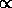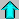# Astronomy 12 - Spring 1999 (S.T. Myers)

## Problem Set #6 (due Thu 25 Mar 1999 5pm)

Last update: 24 March 1999

1. White dwarfs are made mostly of degenerate Carbon. Consider a white dwarf of radius 7000 km, and an effective temperature of 30,000 K. What is the luminosity of this object (in W and in Lsun)?.

If this white dwarf has a mass of 1 Msun, calculate the total internal thermal energy (assume thermal energy of kT per atom). Use this to deduce a cooling time, the time over which it will radiate away its current thermal energy at its current luminosity.

We expect a white dwarf to be formed with an initial temperature equal to that of a (massive) stellar core at the end of triple-alpha, T ~ 10^9 K. Assuming the same radius as above, calculate the luminosity, thermal energy and thus the cooling time.

Here is the new part:

How do these cooling times compare to the age of our galaxy, 1010 years? Set up an equation giving the cooling time as a function of temperature, and use this to find the temperature for which the cooling time of the white dwarf is approximately the age of the galaxy. This is the coolest white dwarf that should be around today.

Note: you will get fairly cool temperature. To really see what is happening, try actually setting up the differential equation

L = dE/dt

where you use the temperature-radius relation for L(T), then the thermal energy for E(T) which gives dT/dt = f(T). This is a polynomial and easy to solve. Do this from an initial time t=0 at Tinit to current time t at T. You will see that the "cooling time" we estimated above is related to the total time it takes to cool to the current temperature T (from the arbitrary much higher Tinit).

2. Consider a neutron star with mass 1.4 Msun and radius of 10 km. Estimate the total gravitational potential (binding) energy of the neutron star.

Gamma ray bursts are events detected by satellites where a ``burst'' of high energy gamma rays are seen over a timescale of less than 1 second. One possibility is that these are caused by the collision of two neutron stars. Assume that the result of this collision is a single neutron star with twice the mass of the original, and calculate the new radius of this object by noting that the mass-radius relationship for a degenerate object

RM-1/3

should hold for neutron stars as well as white dwarfs, as they are supported by degeneracy pressure.

Calculate the binding energy of the merged object, and compare it to the sum of the binding energies of two initial neutron stars. How much energy will be liberated in the collision, assuming only the change in binding energy? Will the new object be a neutron star, or a black hole?

3. Consider a pulsar of mass 1.4 Msun and radius of 10 km, with a rotation period of 0.033 seconds (like the Crab nebula pulsar). The strong magnetic field will cause ionized gas near the neutron star to rotate along with the pulsar. At what distance from the center of the neutron star will the co-rotation velocity equal the speed of light? Note that beyond this radius, known as the light cylinder, matter cannot be induced to co-rotate with the pulsar, and thus defines a limit to the region of strong influence of the neutron star.

As we disussed in class, high-luminosity stars expel material in stellar winds. The system Centaurus X-3 has an O6 primary (mass of 10.5 Msun) and a neutron star secondary (mass of 1.4 Msun, radius 10km, rotation period 4.84 sec) with a binary period of 2.087 days. Compute the orbital radius of the binary, and the radius of the light-cylinder of the neutron star. If the wind is isotropic (uniform and spherically symmetric), then we can assume that the part of the spherical wind that hits the light cylinder (approximate this as a disk of that radius) falls onto the neutron star and releases its energy. Draw a sketch to illustrate the geomtery.

Using a typical O-star mass loss rate of 10^-8 Msun per year, estimate the accretion luminosity of the wind material (in Watts and in Lsun) that we might expect to be emitted in X-rays.

Hint: turn the mass loss rate into a mass flux (kg/s/m^2) at the distance of the neutron star from the O-star, then use the area of the light cylinder (disk) to turn the mass flux into a mass deposition rate (in kg/s), and thus get an energy generation rate = luminosity.Astr12 Index ---Astr12 Home

smyers@nrao.edu Steven T. Myers